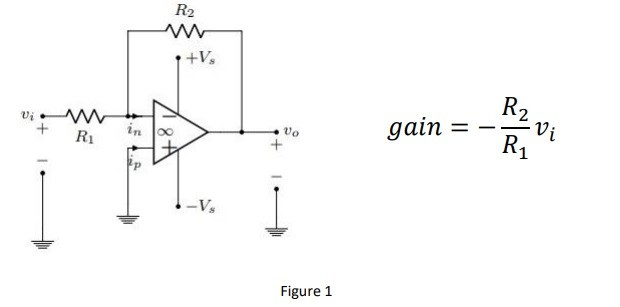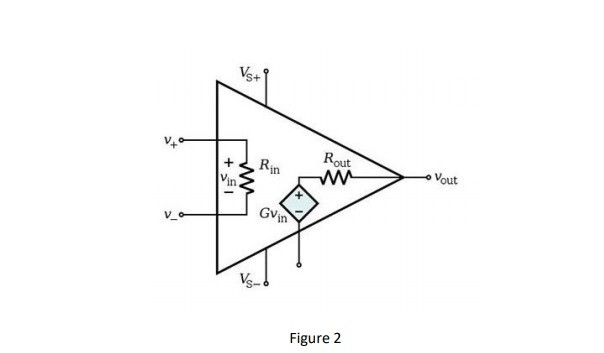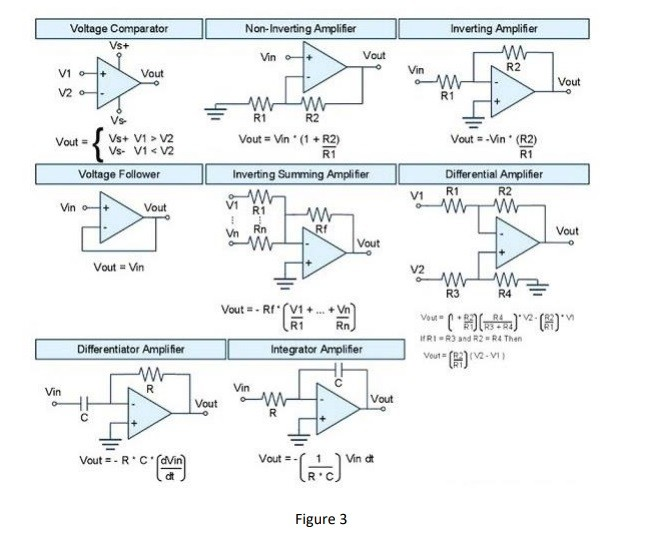op-amps
What is the op-amp
Op-amp or operational amplifier is a high voltage gain electronic signal amplifier. A opamp
have 4 input and 1 output generally. The inputs are inverting input, non-inverting input and
+V source, -V source inputs. It is linear device and using filtering or perform mathematical
operations (add, subtract, integration and differentiation). Multipliers of opamps can change
ambient conditions easly so we should use feedback resistors or capacitors between input
and output. Also we can identify gain with the ratio of feedback resistors. The figure 1 shows
us the connection and formula of gain.This connection called negative feedback and we can protect our circuit from environmental
factors for example; temperature.
Figure 2 shows us the internal structure of opamp. When we idealize the opamp agree the
Rin = ∞ , A=∞ and Rout=0. Also the figure 3 is type of opamps##### Oyun Oynayarak Para Kazanmak İster Misin?

katon1913
18 Ağustos 2019##### Fizyoterapi Okumak

xakgunx
17 Ağustos 2019karhanahmet
17 Ağustos 2019